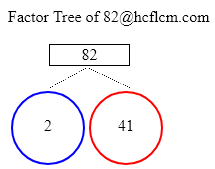# Factor Tree of 82

A factor tree is a type of diagram in which we find the factors of a number and then the factors of those numbers until we can no longer factor them. We can get the factor tree of 82 easily by putting the number 82 in the input box of the Factor Tree Calculator.

Check the different methods to find the factor tree of 82 in the following sections

Factor Tree of:

### Factor Tree of 82 to Calculate the Factors

 82 2 4182 = 2 x 41

Altogether expressing the number in terms of prime factors would be 2 x 41.

### Ways to Find Factors of 82

Here we are providing different methods to find the factors of the number 82. They are splitting numbers and prime factors.

Splitting Numbers

We can divide the number into either of its two factors. In other words, we are looking for the numbers that when multiplied together eqaul 82. Let’s start with 2 x 41 as it results in 82 on multiplying. We will now find the factors or ancestors of split factors, just like in any family tree.

Prime Factors

Let’s take a look at 82 now and we can write as 2 x 41 and place those factors on the tree. similarly to prime numbers obtained in the first step 2, 41 obtained here are also prime numbers and we will end up these branches.

### Factor Tree Calculations

Here are examples of Factor tree calculations.

### FAQ on 82 Factor Tree

1. What is factor tree for 82 ?

Answer: Prime Factors of 82 are 2 x 41.

2. What are the Prime Factors of 82 ?

Answer: Prime Factors of 82 are 2 x 41.